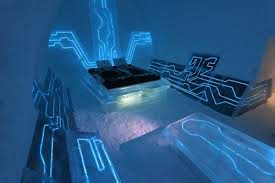Published on Sep 16, 2019

### Abstract

The objective of this project is to determine the Mileage of the vehicle.

### Scope:

Normal existing meter are Analog meter. The one draw back in this meter are we cannot know that exact fuel quantity in the tank. In this project the exact fuel quantity is displayed in the digital form and also speed of the vehicle, how much distance the vehicle has been traveled and further kilometer for available fuel quantity like these information also displayed in the LCD display.

### Brief Methodology:

This project is designed with

• Microcontroller

• Proximity sensor

• Signal conditioning unit

• LCD display

In this project proximity sensor is fixed in the wheel. When the wheel is rotating the proximity sensor gives the pulse to the micro controller. Inside the counter will count that pulse from this we can easily calculate the revolution per minute (RPM). If you multiply into hour we can calculate the revolution per hour by which we can measure speed of the vehicle.

If we know the Diameter of the vehicle we can measure the Distance that the vehicle has been traveled. The fuel storage in the tank is monitoring by float sensor which is fixed in the tank. The float sensor is the special type of sensor in which the resistance value is varied depending upon the liquid level. The variable resistance obtained from the float sensor is converted into variable voltage signal through voltage converter circuit. The converted voltage signal is given into the amplifier in order to amplify the voltage signal. Then the amplified voltage signal is given to ADC.

ADC is nothing but Analog to Digital Converter which converts the analog signal from the float sensor to corresponding digital signal. Then the converted digital signal is given to microcontroller.

Here the microcontroller is the flash type reprogrammable microcontroller in which we have already programmed. After particular distance traveled we can know the fuel quantity is used by the vehicle from the LCD display. So now we knew distance and fuel used by the vehicle from which we can measure the mileage of the vehicle and projected mileage for remaining fuel in the tank. The corresponding information is displayed on the LCD display